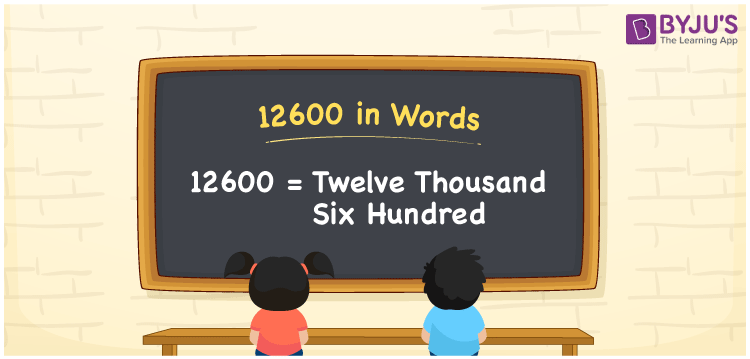# 12600 in Words

12600 in words is written as Twelve thousand six hundred. In both the International System of Numerals and the Indian System of Numerals, 12600 is written as Twelve thousand six hundred. The number 12600 is a Cardinal Number as it represents some quantity. For example, “that smartphone costs 12600 rupees”.

 12600 in words Twelve thousand six hundred Twelve thousand six hundred in numerical form 12600

## 12600 in English Words

12600 in English words is read as “Twelve thousand six hundred.”## How to Write 12600 in Words?

To write 12600 in words, we shall use the place value chart. In the place value chart, put 1 in the ten thousands place, 2 in the thousands place, 6 in the hundreds place and 0 in the tens and the ones place. Let us make a place value chart to write the number 12600 in words.

 Ten Thousands Thousands Hundreds Tens Ones 1 2 6 0 0

Thus, we can write the expanded form as

1 × Ten Thousand + 2 × Thousand + 6 × Hundred + 0 × Ten + 0 × One

= 1 × 10000 + 2 × 1000 + 6 × 100 + 0 × 10 + 0 × 1

= 10000 + 2000 + 600 + 0 + 0

= 12600

= Twelve thousand six hundred.

12600 is a natural number, the successor of 12599 and the predecessor of 12601.

12600 in words – Twelve thousand six hundred

• Is 12600 an odd number? – No
• Is 12600 an even number? – Yes
• Is 12600 a perfect square number? – No
• Is 12600 a perfect cube number? – No
• Is 12600 a prime number? – No
• Is 12600 a composite number? – Yes

## Frequently Asked Questions on 12600 in Words

Q1

### How to write 12600 in words?

12600 in words is written as Twelve thousand six hundred.
Q2

### How to write 12600 in the International and Indian System of Numerals?

In both, the system of numerals, 12600 in words, is written as Twelve thousand six hundred.
Q3

### How to write 12600 in a place value chart?

In the place value chart, write 1 in the ten thousands, 2 in the thousands, 6 in the hundreds and 0 in the tens and the ones, respectively.
Test your Knowledge on 12600 in Words